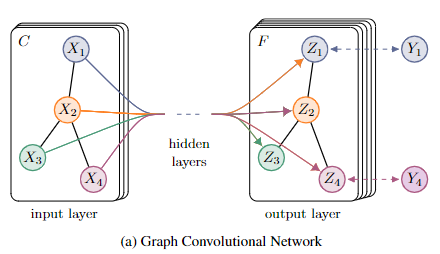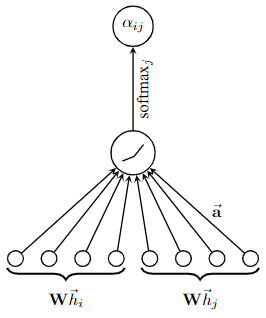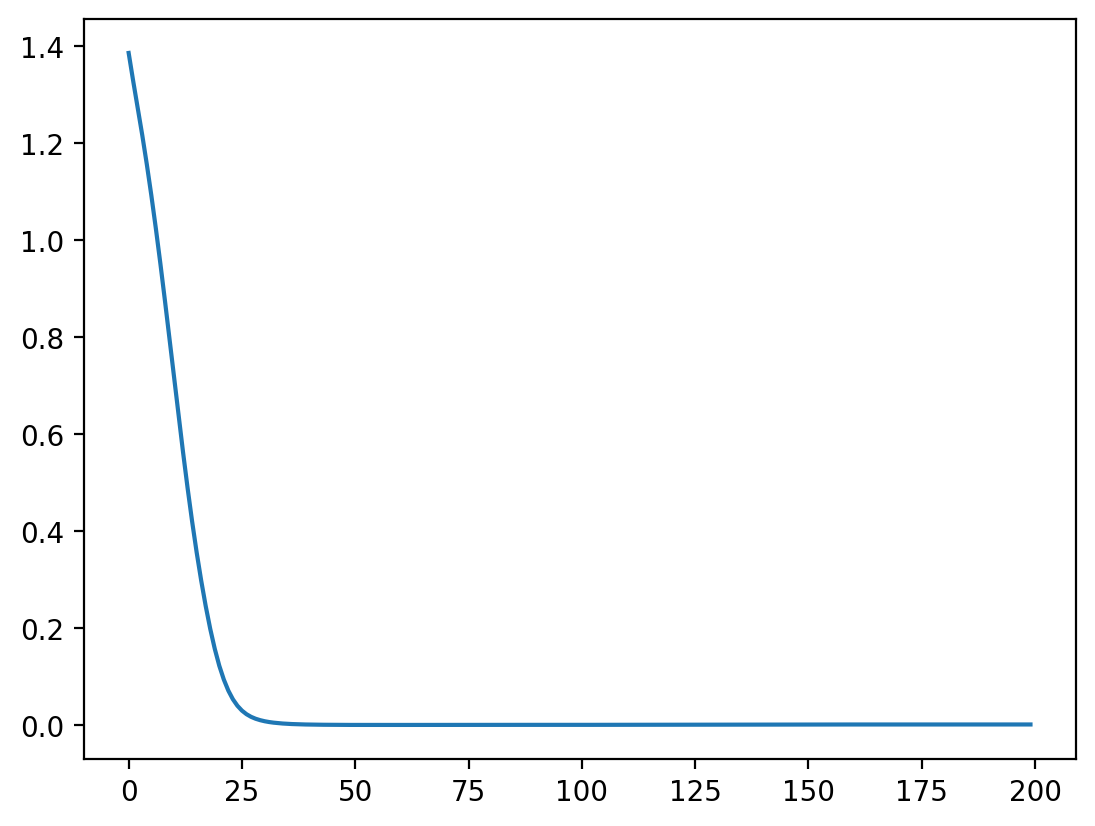V1

2023/05/01阅读：44主题：默认主题

# 浅谈图神经网络

### 基础

#### 1、什么是图

• 节点分类
• 图分类
• 节点聚类：根据连通性将相似的节点分组
• 链接预测：预测缺失链接
• 影响最大化：识别有影响力的节点

#### 2、图神经网络

• 图卷积网络（GCN）和图注意力网络（GAT），两者都涉及传播步骤。传播就是汇集邻居节点和边的信息，来对节点进行更新的过程，在传播的方式有：卷积、注意力机制、门机制、跳跃连接和分层汇聚。不同的传播机制其参数更新方式不同
• 图的空域网络，常用在动态图
• 图的自编码，常用在无监督学习
• 图生成网络， 生成式结构
##### （1）GCN
 论文：Semi-Supervised Classification with Graph Convolutional Networks(ICLR2017) 链接：https://arxiv.org/pdf/1609.02907 源码：https://github.com/tkipf/gcnyellow

GCN是一种频域算法，是目前主流的图神经网络分支，本质上就是以卷积作为传播机制的GNN。下面对其输入数据和传播方式进行介绍。GCN的输入

GCN的传播方式

GCN的层与层之间的传播方式为：

• 为什么要使用

• 表示邻接矩阵，对角线都为0，如果只使用 ，当前节点的特征就会被忽略，所以需要加上一个单位矩阵，让对角线变为1
• 为什么要加入度矩阵？

• 是一个没有经过归一化的矩阵，与特征矩阵相乘时会改变原本的数据分布，所以需要标准化处理。 可以得到一个对称且归一化的矩阵
##### （2）GAT
 论文：Graph Attention Networks(ICLR2018) 链接：https://arxiv.org/pdf/1710.10903 源码：https://github.com/PetarV-/GAT

GAT中的注意力机制是一种mask attention，当前节点只与邻居节点做注意力计算，这样可以保留图的结构信息，并且减少计算量step1：计算注意力系数

step2：加权求和

##### （3）深度图神经网络

（1）当前节点的特征向量=邻接节点的特征向量+连接边的特征向量（这里的+表示拼接，也就是代码中的concat）

（2）根据定义的功能函数 实现特征向量的变换，将变换后的特征向量作为下一层，当前节点的向量表征

### 数据

#### 1、数据调用代码

from torch_geometric.datasets import KarateClubdataset = KarateClub()print(f'Dataset:{dataset}:')print('=' * 30)print(f'Number of graphs:{len(dataset)}')print(f'Number of features:{dataset.num_features}')print(f'Number of classes:{dataset.num_classes}')print('=' * 30)data = dataset# train_mask = [True,False,...] ：代表第1个点是有标签的，第2个点是没标签的，方便后面LOSS的计算print(data)  # Data(x=[节点数, 特征数], edge_index=[2, 边的条数], y=[节点数], train_mask=[节点数])

#### 2、数据可视化

import osfrom torch_geometric.datasets import KarateClubfrom torch_geometric.utils import to_networkximport networkx as nximport matplotlib.pyplot as plt# 画图函数def visualize_graph(G, color):    plt.figure(figsize=(7, 7))    plt.xticks([])    plt.yticks([])    nx.draw_networkx(G, pos=nx.spring_layout(G, seed=42), with_labels=False,                     node_color=color, cmap="Set2")    plt.show()# 画点函数def visualize_embedding(h, color, epoch=None, loss=None):    plt.figure(figsize=(7, 7))    plt.xticks([])    plt.yticks([])    h = h.detach().cpu().numpy()    plt.scatter(h[:, 0], h[:, 1], s=140, c=color, cmap="Set2")    if epoch is not None and loss is not None:        plt.xlabel(f'Epoch:{epoch},Loss:{loss.item():.4f}', fontsize=16)    plt.show()if __name__ == '__main__':    #设置环境变量    os.environ['KMP_DUPLICATE_LIB_OK'] = 'True'    dataset = KarateClub()    print(f'Dataset:{dataset}:')    print('=' * 30)    print(f'Number of graphs:{len(dataset)}')    print(f'Number of features:{dataset.num_features}')    print(f'Number of classes:{dataset.num_classes}')    print('=' * 30)    data = dataset    # train_mask = [True,False,...] ：代表第1个点是有标签的，第2个点是没标签的，方便后面LOSS的计算    print(data)  # Data(x=[节点数, 特征数], edge_index=[2, 边的条数], y=[节点数], train_mask=[节点数])    G = to_networkx(data, to_undirected=True)    visualize_graph(G, color=data.y)

networkx.exception.NetworkXError: random_state_index is incorrect

pip install decorator==5.0.9 

#### 3、如何构建图结构数据集

import torchfrom torch_geometric.data import Datax = torch.tensor([[2, 1], [5, 6], [3, 7], [12, 0]], dtype=torch.float)y = torch.tensor([0, 1, 0, 1], dtype=torch.float)# 定义边edge_index = torch.tensor([[0, 1, 2, 0, 3],  # 起始点                           [1, 0, 1, 3, 2]], dtype=torch.long)  # 终止点# 定义train_masktrain_mask = [(True if d is not None else False) for d in y]# 构建datadata = Data(x=x, y=y, edge_index=edge_index, train_mask=train_mask)print("data:", data)print("train_mask:", data.train_mask)#结果：#data: Data(x=[4, 2], edge_index=[2, 5], y=, train_mask=)#train_mask: [True, True, True, True]

### 代码

#### 1、GNN参数更新

GNN（图神经网络），是一种直接作用于图结构的神经网络，其中 都可以编码成一个特征向量，因此，GNN的本质为特征提取。GNN一个典型的应用就是对图中的节点分类，利用GNN提取。

GNN的训练其实就是对节点向量表征的更新，其实现过程如下：

• 构建图graph
• 初始化特征值embedding
• 初始化聚合函数 。简单的 有拼接、取最大、取平均，复杂的可以后接网络层，如MLP或其他复杂网络
• 遍历图中的节点，对每个节点执行如下操作
• 定义列表root存储当前节点，以及当前节点的父节点（入度节点）
• 根据f，更新embedding中当前节点的向量表征。
• 得到每个节点的向量表征，可以用做后续研究
import numpy as np# 这个图是一个有向无环图(DAG)# -1 代表什么也不连# 图用二维列表，第一行代表节点编号，第二行为对应节点的指向节点，#如：节点0指向节点1和2，也就是说graph为graph的父节点graph = [    [0,0,1,2,3],    [1,2,3,3,4]]# 定义5个节点的初始特征值embeddings = [    [1,2,3],    [2,6,5],    [2,3,7],    [7,8,6],    [1,0,0]]# 定义聚合的权重w全为1f = [1,1,1,1,1]# 下面开始图神经网络的聚合过程（训练过程）# 在这里每个节点只按照方向聚合一层for i in range(len(graph)):  # 每个节点    temp_roots = []#存储当前节点及其父节点    for j, eve in enumerate(graph):        if eve == i:            temp_roots.append(graph[j])    temp_roots.append(i)    around = [        [0, 0, 0],        [0, 0, 0],        [0, 0, 0],        [0, 0, 0],        [0, 0, 0]    ]    # 将temp_roots中的几点对应的around替换成当前的embedding    for every_node_id in temp_roots:        around[every_node_id] = embeddings[every_node_id]    # 开始更新节点i的特征：自己之前的特征+周围节点特征的平均    embeddings[i] = np.matmul(np.array(f),np.array(around))# 输出更新一层后的embeddingsprint(embeddings)

#### 2、GCN代码实现

##### （1）手搓GCN

• 确定输入 和邻接矩阵
• 根据邻接矩阵，计算之前提到的 和度矩阵 ，并对度矩阵求逆 ，计算出 （这个等价于
• 网络层计算
• 定义线性层
• 定义激活函数
• 根据网络层数计算最后输出
import torchimport torch.nn as nnclass GCN(nn.Module):  # GCN模型，向空域的第一个图卷积    def __init__(self, in_c, hid_c, out_c):        super(GCN, self).__init__()  # 表示继承父类的所有属性和方法        self.linear_1 = nn.Linear(in_c, hid_c)  # 定义一个线性层        self.linear_2 = nn.Linear(hid_c, out_c)  # 定义一个线性层        self.act = nn.ReLU()  # 定义激活函数    def forward(self, data, device):        graph_data = data["graph"].to(device)  # [N, N] 邻接矩阵，并且将数据送入设备        graph_data = GCN.process_graph(graph_data)  # 变换邻接矩阵 \hat A = D_{-1/2}*A*D_{-1/2}        flow_x = data["flow_x"].to(device)  # [B, N, H, D]  流量数据        B, N = flow_x.size(0), flow_x.size(1)  # batch_size、节点数        flow_x = flow_x.view(B, N, -1)  # [B, N, H*D] H = 6, D = 1把最后两维缩减到一起了，这个就是把历史时间的特征放一起        # 第一个图卷积层        output_1 = self.linear_1(flow_x)  # [B, N, hid_C],这个就是 WX，其中W是可学习的参数，X是输入的流量数据（就是flow_x）        output_1 = self.act(torch.matmul(graph_data, output_1))  # [B, N, N] ,[B, N, hid_c]，就是 \hat AWX        # 第二个图卷积层        output_2 = self.linear_2(output_1)# WX        output_2 = self.act(torch.matmul(graph_data, output_2))  # [B, N, 1, Out_C] , 就是 \hat AWX        return output_2.unsqueeze(2)  # 第２维的维度扩张    @staticmethod    def process_graph(graph_data):  # 这个就是在原始的邻接矩阵之上，再次变换，也就是\hat A = D_{-1/2}*A*D_{-1/2}        N = graph_data.size(0) # 获得节点的个数        matrix_i = torch.eye(N, dtype=torch.float, device=graph_data.device)  # 定义[N, N]的单位矩阵        graph_data += matrix_i  # [N, N]  ,就是 A+I        degree_matrix = torch.sum(graph_data, dim=1, keepdim=False)  # [N],计算度矩阵，塌陷成向量，其实就是将上面的A+I每行相加        degree_matrix = degree_matrix.pow(-1)  # 计算度矩阵的逆，若为0，-1次方可能计算结果为无穷大的数        degree_matrix[degree_matrix == float("inf")] = 0.  # 让无穷大的数为0        degree_matrix = torch.diag(degree_matrix)  # 转换成对角矩阵        return torch.mm(degree_matrix, graph_data)  # 返回 \hat A=D^(-1) * A ,这个等价于\hat A = D_{-1/2}*A*D_{-1/2}
##### （2）调包实现

import torchimport torch.nn.functional as Ffrom torch_geometric.nn import GCNConvfrom torch_geometric.datasets import KarateClubimport matplotlib.pyplot as pltdataset = KarateClub()class GCN(torch.nn.Module):    def __init__(self):        super(GCN, self).__init__()        #[in_channel,out_channel]与CNN结构一致        self.conv1 = GCNConv(dataset.num_node_features, 16)        self.conv2 = GCNConv(16, dataset.num_classes)    def forward(self, data):        #x表示输入，edge_index表示邻接矩阵        x, edge_index = data.x, data.edge_index        x = self.conv1(x, edge_index)        x = F.relu(x)        x = F.dropout(x, training=self.training)        x = self.conv2(x, edge_index)        #log_softmax+nll_loss==cross_entropy        return F.log_softmax(x, dim=1)device = torch.device('cuda' if torch.cuda.is_available() else 'cpu')model = GCN().to(device)data = dataset.to(device)optimizer = torch.optim.Adam(model.parameters(), lr=0.01, weight_decay=5e-4)model.train()for epoch in range(200):    optimizer.zero_grad()    out = model(data)    loss = F.nll_loss(out[data.train_mask], data.y[data.train_mask])    loss.backward()    optimizer.step()    print('loss:{}'.format(loss.item()))plt.figure()plt.plot(range(len(train_loss)), train_loss)plt.savefig('./图片/图网络训练损失变化.png', dpi=200, bbox_inches='tight')

#### 3、GAT代码实现

##### （1）手搓GAT

GAT的实现包括以下几个步骤：

• 计算
• 计算
• 更新特征向量
class GAT(nn.Module):    def __init__(self, in_features, out_features, dropout, alpha, concat=True):        super(GAT, self).__init__()        self.dropout = dropout        self.in_features = in_features        self.out_features = out_features        self.alpha = alpha        self.concat = concat        self.W = nn.Parameter(torch.empty(size=(in_features, out_features)))        nn.init.xavier_uniform_(self.W.data, gain=1.414)        self.a = nn.Parameter(torch.empty(size=(2*out_features, 1)))        nn.init.xavier_uniform_(self.a.data, gain=1.414)        self.leakyrelu = nn.LeakyReLU(self.alpha)    def forward(self, h, adj):        Wh = torch.mm(h, self.W) # h.shape: (N, in_features), Wh.shape: (N, out_features)        e = self._prepare_attentional_mechanism_input(Wh)        zero_vec = -9e15*torch.ones_like(e)        attention = torch.where(adj > 0, e, zero_vec)        attention = F.softmax(attention, dim=1)        attention = F.dropout(attention, self.dropout, training=self.training)        h_prime = torch.matmul(attention, Wh)        if self.concat:            return F.elu(h_prime)        else:            return h_prime        def _prepare_attentional_mechanism_input(self, Wh):        #计算$e_{ij}$        # Wh.shape (N, out_feature)        # self.a.shape (2 * out_feature, 1)        # Wh1&2.shape (N, 1)        # e.shape (N, N)        Wh1 = torch.matmul(Wh, self.a[:self.out_features, :])        Wh2 = torch.matmul(Wh, self.a[self.out_features:, :])        # broadcast add        e = Wh1 + Wh2.T        return self.leakyrelu(e)x = torch.randn(6,10)adj=torch.tensor([[0,1,1,0,0,0],                  [1,0,1,0,0,0],                  [1,1,0,1,0,0],                  [0,0,1,0,1,1],                  [0,0,0,1,0,0,],                  [0,0,0,1,1,0]])my_gat = GAT(10,5,0.2,0.2)print(my_gat(x,adj))#输出#tensor([[-0.9736,  3.7097,  1.1458,  0.1472,  0.3813],#        [-0.7384,  1.8524,  0.2285,  2.0530,  1.8134],#        [-0.4622,  0.6023,  0.1506,  0.0892,  0.0965],#        [ 1.1016,  1.4553, -0.6057,  1.0181,  1.9374],#        [-0.5572,  4.4485,  0.1592, -0.5805, -0.0197],#        [ 0.0000,  0.0000,  0.0000,  0.0000,  0.0000]], grad#_fn=<EluBackward0>)

GAT在实现过程中也使用到了多头注意力机制，用以增加特征多样性，增强模型拟合能力

class GAT_Mutil_Head(nn.Module):    def __init__(self, nfeat, nhid, nclass, dropout, alpha, nheads):        """Dense version of GAT."""        super(GAT_Mutil_Head, self).__init__()        self.dropout = dropout        # 加入Multi-head机制        self.attentions = [GAT(nfeat, nhid, dropout=dropout, alpha=alpha, concat=True) for _ in range(nheads)]        for i, attention in enumerate(self.attentions):            self.add_module('attention_{}'.format(i), attention)        self.out_att = GAT(nhid * nheads, nclass, dropout=dropout, alpha=alpha, concat=False)    def forward(self, x, adj):        x = F.dropout(x, self.dropout, training=self.training)        x = torch.cat([att(x, adj) for att in self.attentions], dim=1)        x = F.dropout(x, self.dropout, training=self.training)        x = F.elu(self.out_att(x, adj))        return F.log_softmax(x, dim=1)
##### （2）调包实现

class GAT(torch.nn.Module):    def __init__(self, in_c, hid_c, out_c):        super(GAT, self).__init__()        self.GATConv1 = GATConv(in_channels=in_c, out_channels=hid_c)        self.GATConv2 = GATConv(in_channels=hid_c, out_channels=out_c)    def forward(self, data):        x = data.x        edge_index = data.edge_index        hid = self.GATConv1(x=x, edge_index=edge_index)        hid = F.relu(hid)        out = self.GATConv2(hid, edge_index=edge_index)        return F.log_softmax(out, dim=1)可以看到，相比于GCN，GAT的收敛速度更快、更平滑。

### 总结

GCN与GAT的本质都是将邻居节点的特征聚合到中心顶点上，再学习新的顶点的特征表示

GCN

• 基于频域，利用了拉普拉斯矩阵，基本思想是利用图卷积对节点进行特征表示

• 可以堆叠多层卷积来提高效果，不过不是越多越好，一般2-3层的效果最好

• 可以学习图的局部结构

• GCN是一种全图计算方式，无法处理动态图，将局部的图结构和节点特征结合，但这种方式和图的结构联系紧密，使得模型泛化性不足

• GCN利用了对称的拉普拉斯矩阵，所以其假设图是无向的，不能直接用于有向图

• 不能为每个邻居分配不同的权重

• 处理大规模图数据时，需要大量的计算资源，因为模型中存在对矩阵的逆的求解

GAT

• 用注意力机制对邻近节点特征加权求和，邻近节点特征的权重完全取决于节点本身，独立于图结构，泛化性强

• 由于使用了注意力机制，所以可以自适应的学习每个节点与其邻居节点之间的关系

• 能够有效处理稀疏图，因为只考虑每个节点的邻居

• 由于注意力机制的存在，计算复杂度高

• 对孤立节点不友好

【参考文章】

CSDN: GNN的理解与研究, 2022

CSDN: 代码+通俗理解图神经网络GNN, 2021

CSDN: 图神经网络（GNN）模型原理及应用综述, 2023

CSDN: PyTorch搭建图卷积神经网络（GCN）完成对论文分类及预测实战（附源码和数据集）, 2022

CSDN: 图注意网络GAT理解及Pytorch代码实现【PyGAT代码详细注释】, 2023

Distill: A Gentle Introduction to Graph Neural Networks, 2021

Distill: Understanding Convolutions on Graphs, 2021

Kipf T N, Welling M. Semi-supervised classification with graph convolutional networks[J]. arXiv preprint arXiv:1609.02907, 2016.

Veličković P, Cucurull G, Casanova A, et al. Graph attention networks[J]. arXiv preprint arXiv:1710.10903, 2017.

github.io: 图神经网络, 2019

github: GCN_predict-Pytorch, 2020

简书: 【图结构】之图注意力网络GAT详解以及GAT的推广, 2020V1